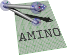amino  1.0-beta2 Lightweight Robot Utility Library
plot.h File Reference

Go to the source code of this file.

## Classes

struct  aa_plot_opts
options for a plot More...

## Typedefs

typedef struct aa_plot_opts aa_plot_opts_t
options for a plot

## Functions

AA_API void aa_plot_row_series (size_t m, size_t n, double *t, size_t inct, double *Y, size_t ldY, const struct aa_plot_opts *opts)
Plots Y over t. More...

## ◆ aa_plot_row_series()

 AA_API void aa_plot_row_series ( size_t m, size_t n, double * t, size_t inct, double * Y, size_t ldY, const struct aa_plot_opts * opts )

Plots Y over t.

Parameters
 m rows in Y n columns in Y, length of t t $$t \in \Re^n$$ vector of times for x-axis inct increment of t Y $$Y \in \Re^m\times\Re^n$$ Matrix of y-axis values. Each column is a data point, rows are the indepedent series. opts plot options